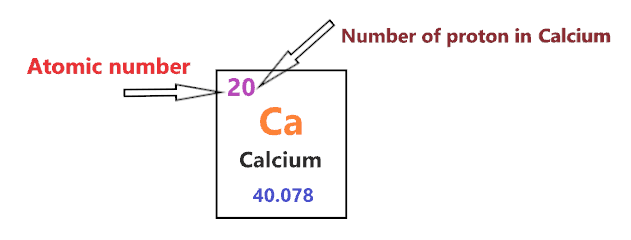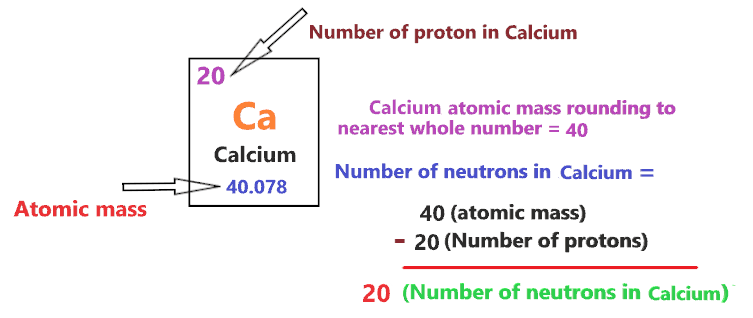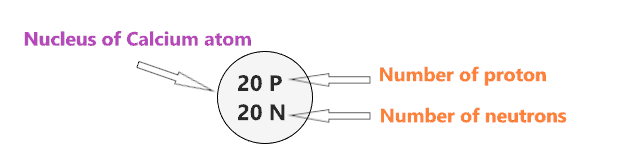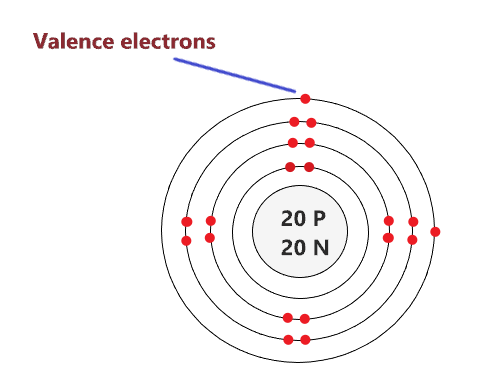# Bohr model of Calcium atom - How to draw Calcium(Ca) Bohr-Rutherford diagram?

Home  > Chemistry Article > Calcium Bohr model

The Bohr Model of Calcium(Ca) has a nucleus that contains 20 neutrons and 20 protons. This nucleus is surrounded by four-electron shells named K-shell, L-shell, M-shell, and N-shell. The outermost shell in the Bohr diagram of Calcium contains only 2 electrons that also called valence electrons.

 Name Calcium Bohr Model Number of neutrons 20 Number of protons 20 Number of electrons 20 Total electron shells 4 Electron in the First shell(K) 2 Electrons in the Second shell(L) 8 Electrons in the Third shell(M) 8 Electrons in the Fourth shell(N) 2 Total valence electrons in Calcium 2
Page Contents

## How to draw Bohr Model of Calcium(Ca)?

Bohr model describes the visual representation of orbiting electrons around the small nucleus. It used different electron shells such as K, L, M, N…so on. These shells hold a specific number of electrons, the electron shell which is closest to the nucleus has less energy and the electron shell which is farthest from the nucleus has more energy.

Bohr diagram is very interesting and easy to draw. Here, we will draw the Bohr diagram of the Calcium atom with some simple steps.

## Steps to draw the Bohr Model of Calcium atom

1. Find the number of protons, electrons, and neutrons in the Calcium

Protons are the positively charged particles and neutrons are the uncharged particles, both these are constituents of the atom nuclei. Electrons are the negatively charged particles that orbit the nucleus of an atom

To find the number of protons an atom contains, just look at their atomic number.

If the atomic number of atom is 45, then proton will also be 45, if atomic number is 46, then proton will also be 46.

So, the atomic number for Calcium is 20, hence, the number of protons in the Calcium atom is also 20.Now, to determine the number of neutrons in an atom, use this formula.

⇒ Number of neutrons in atom = Atomic mass of the atom(rounded to the nearest whole number) – Number of proton in an atom

For example, An atom have 33.988 atomic mass and 16 protons.

Then, to find the number of neutron, round the atomic mass to the near whole number, so, atomic mass 33.988 round to 34.

= (34 – 16 protons) = 18 number of neutrons in the atom

Now, To get the number of neutrons in a Calcium atom, look at its atomic mass which is 40.078 rounded to 40, and the number of protons in Calcium is 20.

∴ Hence, the number of neutrons in Calcium atom = (40 – 20) = 20It should be noted that “The number of electrons in a neutral atom is equal to the number of protons”.

So, the Calcium atom is neutral, hence, its number of electrons will be equal to the number of protons which is 20 as we already discussed.

⇒ The number of electrons in a Calcium atom = 20

⇒ The number of protons in Calcium atom = 20

⇒ The number of neutrons in a Calcium atom = 20

2. Draw the nucleus of an atom

A nucleus is a dense and small region that contains the number of protons and neutrons of an atom.

In this step, we have to draw a small circle that consists of a number of protons and the number of neutrons of a Calcium atom.3. Draw the First electron shell

“An electron shell may be thought of as an orbit followed by electrons around an atom’s nucleus.”

The first electron shell is also called the K-shell, this is the closest shell to the nucleus of an atom and can hold a maximum of two electrons.

As we know, the Calcium atom has a total of 20 electrons. So, put two electrons from it, in the first shell, next to each other.We have successfully drawn the first shell of the Calcium atom that can hold 2 electrons. As a Calcium atom has a total of 20 electrons, and from 20 electrons we have used two electrons in the first shell.

∴ (20 – 2) = 18 electrons

Therefore, we are left with 18 electrons, let’s put them in the next shells of the Calcium atom.

4. Draw the Second electron shell

The second shell also called the L-shell that can hold a maximum of 8 electrons. This shell is drawn after the first electron shell.

In the second electron shell, the electrons are added one at a time, starting from the top position then going in a clockwise direction.

In second shell, electrons are added one at a time in clockwise direction as a clock position – 12 o’clock, 3 o’clock, 6 o’clock, 9 o’clock positions.Once you place the electrons one at a time to each of the four sides(Top – Right – Bottom – Left], start pairing or doubling them.

So, we have 18 remaining electrons of a Calcium atom, and the 2nd shell can only hold a maximum of 8 electrons.

Therefore, put the 8 electrons of the Calcium atom in the 2nd electron shell, start from the top position, put electrons one at a time, and go in a clockwise direction(Top – Right – Bottom – Left). And, finally, pair them up.As we have a total of 20 electrons for the Calcium atom and we placed 2 electrons in the first shell and 8 electrons in the second shell, hence, we are left with 10 electrons more.

Let’s place it in the next shells.

5. Draw the Third electron shell

The third shell is also called the M-shell and it has the capacity to hold up to 18 electrons but, for the elements in the first few periods, the third shell only holds up to 8 electrons.

“The third shell can be considered to hold 8 or 18 electrons but in total the third shell can hold 18 electrons.”

For the first few elements, say, till atomic number 20 on the periodic table, the third shell holds up to 8 electrons.

⇒ For atomic number 20 or less than 20, the third shell can hold up to maximum of 8 electrons.

⇒ For the atomic number more than 20, say, atomic numbers 21, 22, 23, 24, 25, 26, 27,…….etc., the third shell is filled with more than 8 electrons and up to maximum of 18 electrons.

For the Calcium atom, the third shell is filled with the same procedure as the second shell and it will hold up to 8 electrons as its atomic number is 20.

So, we have left with 10 electrons of a Calcium atom and the third shell will hold up to a maximum of 8 electrons.

Therefore, put the 8 electrons of the Calcium atom in the 3rd electron shell, start from the top position, put electrons one at a time, and go in a clockwise direction(Top – Right – Bottom – Left). And, finally, pair them up.Now we are just left with only 2 electrons more, let’s put it in the fourth shell.

6. Draw the Fourth electron shell

Here, draw the fourth electron shell and put the remaining two electrons of Calcium, starting from the top position and going in a clockwise direction.### Bohr model of the Calcium atom

That’s all, this is our Bohr model of the Calcium atom that contains 20 protons and 20 neutrons in the nucleus region, and 20 electrons are orbited around the nucleus, two electrons in the first shell, eight electrons in the second shell, eight electrons in the third shell, and two electrons in the fourth shell.

## Find Valence electron of Calcium through its Bohr diagram

Valence electrons are found in the outermost shell of an atom and they can take participate in the formation of a chemical bond. These electrons have more energy compare to the inner shell electrons.

From the Bohr diagram of an atom, we can easily find the number of valence electrons in an atom by looking at its outermost shell.

So, we have to find a valence electron in the Calcium atom, for this, look at its Bohr diagram.

Bohr’s diagram of Calcium has four electron shells (K, L, M, N), the inner shell is K-shell and the outermost shell is N-shell.

Hence, the electrons found in the N-shell of the Calcium atom are its valence electrons because it is the outermost shell that also called the valence shell.

The N-shell or outer shell of the Calcium Bohr model contains only 2 electrons, therefore, the number of the valence electrons in the Calcium atom is also 2.## Electron dot diagram of a Calcium atom

Electron dot diagram also called lewis structure which represents the valence electrons of atoms.

As, from the Bohr diagram of Calcium, we got to know, it has only 2 valence electrons.

So, just represent the 2 valence electron around the Calcium atom as a dot.## The electron configuration of Calcium

Calcium has an atomic number of 20 and it contains a total number of 20 electrons. From the Bohr model of Calcium, we know, 2 electrons are in the K-shell, 8 electrons are in the L-shell, 8 electrons are in the M-shell and 2 electrons are in the N-shell.

So, based on the shell, the electronic configuration of the Calcium atom is [2, 8, 8, 2].

Or the electronic configuration of Calcium is 1s22s22p63s23p64s2 since it contains a total of 20 electrons.

## FAQ

### How many electron shells a Calcium Bohr model contains?

Electron shell also called energy level, you can find the number of electron shells for an element by knowing its period number in the periodic table.

The elements or atoms in the first period of the periodic table have one energy level or one electron shell, same as, the elements in the second period have two energy levels or two-electron shells and so on…

So, the Calcium atom belongs to the 4th Period in the periodic table, hence, the number of electron shells for the Bohr model of Calcium will be 4 (K-shell, L-shell, M-shell, and N-shell).

### What is the outer shell of the Bohr diagram of the Calcium atom?

The outermost shell also called the valence shell, which contains valence electrons of the atom.

According to the Bohr diagram of Calcium, the outer shell is N-shell which contains only two valence electrons.

## Properties of Calcium

1. It appears as dull gray, silver; with a pale yellow tint.
2. It has a boiling point of 1484 °C and a melting point of 842 °C.
3. It has an FCC crystal structure.
4. It is the fifth most abundant element in Earth’s crust.
5. It can form a dark oxide-nitride layer when exposed to air because of its reactive nature.

## Summary

• The Bohr model of Calcium(Ca) is drawn with four electron shells, the first shell contains 2 electrons, the second shell contains 8 electrons, the third shell contains 8 electrons and the fourth shell contains 2 electrons.
• Calcium is neutral and its atomic number is 20, hence, the number of protons and electrons available for its Bohr diagram is also 20.
• The number of neutrons for the Bohr diagram of Calcium can be found by subtracting the number of protons from the atomic mass(rounded to the nearest whole).
• The electron configuration of Calcium in terms of the shell is [2, 8, 8, 2], or in normal form, it is [Ar] 4s2.
##### Subscribe to Blog via Email

Join 2 other subscribers

Share it...